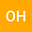Hyperbolic Tangent Ansatz Method to Partial Differential Equations by Using Symbolic Computation
•• Ozlem Ersoy Hepson
Ozlem Ersoy Hepson
Eskisehir Osmangazi Universitesi Fen Edebiyat Fakultesi

Corresponding Author:[email protected]

Author Profile## Abstract

Space--time fractional forms of the modified Korteweg-de Vries equation, the modified Equal Width equation and Benney-Luke equations are solved by using simple hyperbolic tangent ansatz approach. A simple compatible wave transformation in one dimension is employed to reduce the governing equations to integer--ordered ODEs. Then, the hyperbolic tangent ansatz is used to derive exact solutions. Some illustrative examples are presented for some particular choices of parameters and derivative orders.